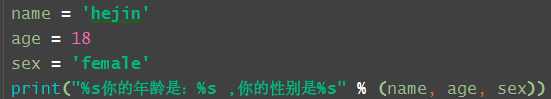﻿ 详解Python数据类型、进制转换、字符串格式化的问题_python_脚本之家
python# 详解Python数据类型、进制转换、字符串格式化的问题

## 1. 整数、浮点数和复数Python2中区分整型int、长整型long
Python3中只有统称为 整型int

### 浮点数float组成： 由整数位，小数点，小数位组成，也可以用科学计数法表示### Decimal

```from decimal import Decimal  #从decimal 包导入Decimal  类
mydec = Decimal("3.22")   #将字符串转换成decimal类型
print(mydec, type(mydec))
mydec2 = Decimal(3.22)   #浮点型3.22 是不精确的，转换成decimal就是精确的
print(mydec2, type(mydec))

3.220000000000000195399252334027551114559173583984375 <class 'decimal.Decimal'>```

### 复数

dir（a） 查看属性
a.imag 获取虚部，无论定义的时候是整型还是浮点型最终都会转换成浮点型
a.real 获取实部

```>>> a = 4 + 5j
>>> a.imag
5.0
>>> a.real
4.0```

## 2.进制转换### 进制数字类型

```>>> a=0b11  二进制   binary
>>> type(a)
<class 'int'>
>>> b=0o11  八进制 octonary
>>> type(b)
<class 'int'>
>>> c=0x11 十六制   hexadecimal
>>> type(c)
<class 'int'> ```

### 整型进制操作

```>>> num = 100
>>> bin(num)  整型转换成二进制，()里面只能是整型
任意进制转2进制, 接收一个int, 返回一个str
'0b1100100'
>>> oct(num) 任意进制转8进制, 接收一个int, 返回一个str
'0o144'
>>> hex(num)   任意进制转16进制, 接收一个int, 返回一个str
'0x64'```
```>>> int (0o11) 接收整型
9
>>> int("0o11",base=8) 接收整型，但要指定几进制
9
>>> int("11")  接收整型，可以不指定，因为它是一个数字
11```## 3. 字符串

### 3.1 转义字符

r标志：是输出原始字符串，不转义### 3.2 字符串取值

```>>> a = 'abcdefghijklmnopq'
>>> a
'a'
>>> a[-8]
'j'```

```str[start:end:step]       左闭右开
step 为正数 从左至右
step 为负数 从右至左
str[2:]  从左至右 第三个数开始
str[:7] 从左至右到第七位
str[::-1]  从右至左 步长为1
str[4::-2]  从第五个数开始从右至左 步长为2```
```>>> a = 'abcdefghijklmnopq'  一旦定义不可改变
>>> a[2:5]
'cde'```

## 4. 字符串运算与转换

```>>> str1="qqq"
>>> str2 = "lll"
>>> str1+str2 # 字符串拼接
'qqqlll'
>>> str3="abc"
>>> str3*3
'abcabcabc'字符串复制
>>> result = str(100)  # int转换成字符串
>>> type(result)
<class 'str'>
>>> result
'100'```

## 5. 字符串的常用方法

### 判断系列```>>> str7= "helloabc xx zz"
>>> str7.startswith("abc")
False
>>> str7.startswith("abc",5)
True
>>> str7.startswith("abc",5,7)
False
>>> str7.startswith("abc",5,8)
True```

### 查找统计系列len不是字符串的属性方法，不能通过.len的方式去计算长度

### 字符串转换类```>>> str7
'helloabc xx zz'
>>> str7.upper()  转大写
'HELLOABC XX ZZ'
>>> str7.title()  转标题格式
'Helloabc Xx Zz'
>>> str9 = str7.title().swapcase()  大小写互换
>>> str9
'hELLOABC xX zZ'
>>> str9 = "a b c d e f"
>>> str9.split()  默认按空格切割
['a', 'b', 'c', 'd', 'e', 'f']
>>> str10 = "a#b#c#d#e#f#g#h"
>>> str10.split("#")
['a', 'b', 'c', 'd', 'e', 'f', 'g', 'h']
>>> str11 = str10.split("#")
>>> str11
['a', 'b', 'c', 'd', 'e', 'f', 'g', 'h']
>>> "*".join(str11)
'a*b*c*d*e*f*g*h'```### +和join的效率问题

+ 拼接效率比join低。+拼接，每一次出现都会在内存中开辟一个新的空间，所以效率低## 6 . 字符串格式化

### %格式化• (name):命名
• flags: +,-,’ '或0。

+表示右对齐；-表示左对齐；
’ '为一个空格，表示在正数的左侧填充一个空格，从而与负数对齐，0表示使用0填充。

• width表示显示宽度
• precision表示小数点后精度

%s 一个萝卜一个坑，一一对应，不能多也不能少### format格式化

```num1 = 0.1416926   #转换成百分位
print("%.2f%% " % (num1 * 100))```### f格式化

python2不支持这种写法

```print(f"my name is {name}，my age is {age}")

print(f"my name is {name}，my age is {age:*>10}")

### 练习

```a = "字符串拼接1"
b = "字符串拼接2"
print("方式1：" + a + b)
print("方式2：%s%s" % (a, b))
print(f"方式3：{a}{b}")
print("方式4：{0}{1}".format(a, b))
c = a + b
print("新拼接的字符串长度为：", len(c))
print("第七个字符为：", c)
str1 = "8*y*cali*china**it*soft*linux*python"
count = str1.count("*")
list = str1.split("*")
str2 = "".join(list)
print("*" * count + str2)```

```str1 = "8*y*cali*china**it*soft*linux*python"
count = str1.count("*")
list = str1.split("*")
str2 = "".join(list)
print("*" * count + str2)```

## 7. 字符串拼接

print(“%s %s”%(str1,str2))

```>>> str1 = "你好"
>>> str2 = "hejin"
>>> str1+str2    #方式1:加号拼接
'你好hejin'
>>> "".join([str1,str2])  #方式2:join拼接
'你好hejin'
>>> print("%s,%s"%(str1,str2))  #方式3:% 占位符

>>> "{},{}".format(str1,str2)   #方式4:format()
'你好,hejin'
>>> "{0},{1}".format(str1,str2) # 方式5
'你好,hejin'
>>> "{1},{0}".format(str1,str2)
'hejin,你好'
>>> "{a},{b}".format(a=str1,b=str2)  # 方式6
'你好,hejin'
>>> "{b},{a}".format(a=str1,b=str2)
'hejin,你好'
>>> f"{str1},{str2}"  # 方式7：f格式化
'你好,hejin'```

```嫌疑人A、B、C、D的笔录如下，其中三人为真，一人为假：
a:我不是小偷
b:C是小偷
c:小偷肯定是D
d:小偷肯定是D```
```lst = ["a", "b", "c", "d"]
for x in lst:
if ("a" != x) + ("c" == x) + ("d" == x) + ("d" != x) == 3:
print(f"{x}是小偷")```